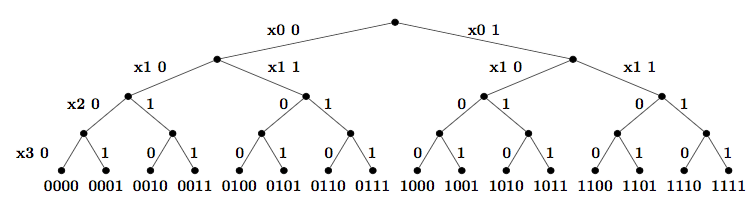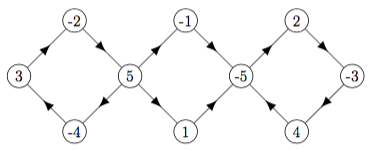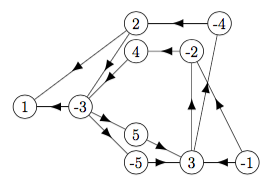# coping with NP-C

exhaustive search   backtrack   sat solver   2-sat   phase transition

## exhaustive tree search (ETS)

• common form of exhaustive search: traverse a decision tree

• tree search: traversal of search space based on a decision tree

• e.g. sat: choice 0/1 for each boolean variable

• left branch of node at depth k: variable k = 0

• left branch of node at depth k: variable k = 1

• usually, depth first traversal

• in memory, only need path from root to current node

• example below for n = 4, variables x1 x2 x3 x4

example: tree search of sat instance, n=4## intelligent ETS: backtracking

how to make ETS intelligent ?
• with no improvements, WC, whole tree traversed

• how to avoid searching whole tree?

• backtrack when current node cannot extend to solution

• example below for n = 4, variables x0 x1 x2 x3

• formula [0 1 2 3] [0 -1] [1 -2] [2 -3]

• once variable x is set

• if clause containing x sat, remove clause from formula

• if clause containing x not sat, remove variable from clause

• once clause becomes empty [ ], formula is unsat

• once formula becomes empty φ, formula is sat

## sat solvers

example: backtrack sat solvermore intelligent exhaustive search
• use current partial solution to pick next search step

more intelligent sat solver
• use current reduced formula to pick next variable to set

• e.g. if some clause has size 1, set variable so clause sat

• more generally: set variable from any shortest clause

• improving sat solvers is continuing research area

• classic sat solver DPLL

• unit propagation

• pure literal elimination

• best sat solvers still wc exptime

• below: simple sat solver

• set variable from any shortest clause

• backtrack once some clause unsat

simple backtrack sat solver
```def sat(f): return (True if len(f)==0 else False)
def unsat(a): return (True if a==UNSAT else False)

def backsat(f,a):
if unsat(a) or sat(f): return f,a
minj = f.index(min(f,key=len))  # clause with fewest literals
if len(f[minj])==0:
a = UNSAT
return f,a
if len(f[minj])==1:
fixliteral(f[minj], f, a)
return backsat(f,a)
#split: 2 possible bool. vals for literal f[minj]
fcopy, acopy = mycopy(f,a)
fixliteral(f[minj], f, a)  # f[minj] True
f,a = backsat(f,a)
if sat(f): return f, a
f,a = fcopy, acopy
fixliteral(-f[minj], f, a) # f[minj] False
return backsat(f, a)
```
time?
• above solves random n, k, m = 30, 5, 600 instance in few seconds, while brute force takes much longer (why?)

restricted instances can be easier
• e.g. knapsack, each value/wt has O(logk n) bits, polytime

• e.g. 2-sat?

• f1 = [[1,-5],[-2,-3],[3,4],[-4,-5],[2,5],[-1,-5]] satisfiable ?

• f2 = [[1,-2],[1,3],[-2,-3],[2,4],[-3,-4],[3,-5],[3,5]] satisfiable ?

## polytime 2-sat via implication digraph

implication digraph of 2-sat formula
• one node for each literal

• for each clause [a,b]

• draw arc from literal negate(a) to literal b

• draw arc from literal negate(b) to literal a

• e.g. formula f1 below

• nodes (1) (2) (3) (4) (5) (-1) (-2) (-3) (-4) (-5)

• clause [1 -5]   (-1) ----> (-5)       (1) <---- (5)

• clause [-2 -3]   (2) ----> (-3)       (-2) <---- (3)

• ...

f1 [1 -5] [-2 -3] [3 4] [-4 -5] [2 5] [-1 -5]f2 [1 -2] [1 3] [-2 -3] [2 4] [-3 -4] [3 -5] [3 5]2-sat in P
• n variables, m clauses

• construct implication digraph D

• if some scc of D contains literal x and -x then unsat (why ?)

• otherwise

• consider sink scc S of D

• assign literals according to S (no inconsistencies: why ?)

• remove S, and all variables in S, from D

• continue with remaining digraph D'

• this algorithm takes polytime (why ?)

• this algorithm correct (why ?)

example f1
• f1 has sccs {-2 5 -4 3} {-1} {1} {2 -3 4 -5}

• no scc contains both x and -x, so f1 satisfiable

• sink scc {2 -3 4 -5} so set 2T 3F 4T 5F

• from digraph, remove this scc and all instances of variables 2 3 4 5

• remaining digraph has sccs {-1} {1}

• each is sink scc, so pick either, assign 1F or 1T

example f2
• f1 has sccs {-1} {-2 2 -3 3 -4 4 -5 5} {1}

• some scc contain both x and -x, so f2 unsatisfiable

## 3-sat phase transition

• if clause/variable ratio m/n small, say ≤ 3

• Prob(sat) close to 1

• easy to solve

• if clause/variable ratio m/n big, say ≥ 5

• Prob(sat) close to 0

• easy to solve

• if clause/variable ratio m/n ≈ 4.2

• Prob(sat) close to .5

• hardest to solve

• see p3

• easy-hard-easy common to NP-C